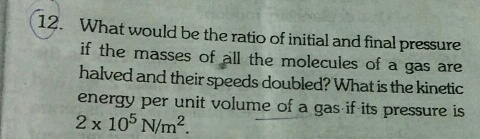# What would be the radio of initial and final pressure if the masses of all the molecules of a gas are halved and their speeds doubled? What is the kinetic energy per unit volume of a gas if tis pressure is 2×105 N/m24 years ago
Dear Yash
From the Kinetic Gas equation:

PV=1/3(nMv2) —————— (1)

If the mass is divided by 2, then:

PV=1/6(nMv2)

If the velocity is multiplied by 2, then:

PV=1/6(nM * (2v)2)

Therefore:

PV=1/6 * 4(nMv2)

Finally:

PV=2/3(nMv2) —————— (2)

From equation (2), the final pressure is:

2/3V (nMv2)

And the initial pressure, from equation(1), is:

1/3V (nMv2)

Therefore:

P1/P2= 1/3 divided by 1/6

Which is 2.

Regards